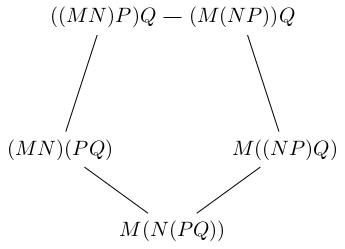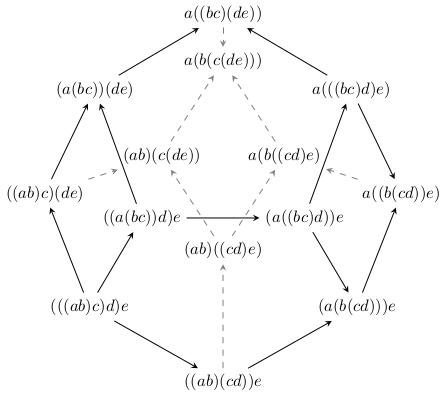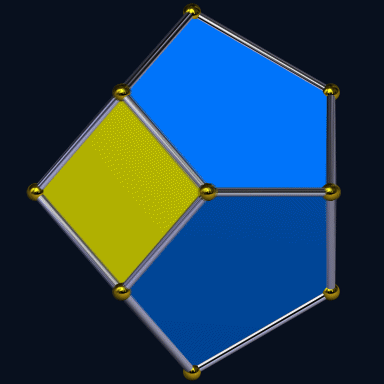# Associahedra

[2019-04-17 Wed]

I had a fun conversation about associativity the other day with one of our first year students who is taking my linear algebra class. This blog post is inspired by that conversation.

Mathematicians are big on proofs. We like to work out every tiny detail of an argument before presenting it to the world. Sometimes this can seem like pedantry, and doubtless there are mathematicians who are pedants. But I would like to argue that this process of carefully examining the minutiae of an argument can lead one to new and unexpected discoveries. This is completely analogous to the way that a scientist, confused by discrepancies between theory and experiment, can find a new, deeper theory by careful and imaginative examination of the basic assumptions of the old theory.

I'm going to introduce a mathematical idea which will seem, at first sight, pretty boring. Then I'll show you how it leads you naturally to something completely unexpected.

Associativity is the property of multiplication which can be expressed as $$(AB)C=A(BC)$$. For example, if you have numbers 2, 3 and 5 and you want to compute 2 times 3 times 5, it doesn't matter if you first compute $$2\times 3=6$$ and then $$6\times 5=30$$ or if you first compute $$3\times 5=15$$ and then $$2\times 15=30$$.

In other words, the way you bracket your multiplications doesn't matter. So you can be excused for writing $$2\times 3\times 5$$ with no brackets to tell you in what order you should be multiplying things. Note that this is a different issue from commutativity, which is the statement that $$AB=BA$$ (e.g. $$2\times 3=3\times 2$$).

Associativity is one of the key properties of multiplication which we use everywhere in mathematics on a daily basis without noticing. Often, mathematicians will prove theorems about algebraic structures which only satisfy associativity and a handful of other properties.

Now, if bracketing really doesn't matter then it shouldn't matter in longer expressions. For example you would hope that $$((MN)P)Q=M((NP)Q)$$. Happily, all such bracketings are equivalent by a finite sequence of "associativity moves": \begin{align*} ((MN)P)Q&=(MN)(PQ)\\ &=M(N(PQ))\\ &=M((NP)Q) \end{align*} At each step in this calculation we just used ordinary associativity. For example, the step: $((MN)P)Q=(MN)(PQ)$ is simply $$(AB)C=A(BC)$$ with $$A=MN$$, $$B=P$$ and $$C=Q$$.

Most people who have followed this far will be happy to assume that any rebracketing can be handled this way. But some will want a proof. Though we are straying into the realms of pedantry, some beautiful and unexpected structures will emerge when we try to prove this, so it is worth taking time to think about it. Indeed, this is true of much mathematics: by trying to fill in all the gory details and justify every step of the way you are led to discover complicated and beautiful structures hiding below the surface which you would just miss completely if you just said "screw it, let's just assume it's true".

Let's try and prove that every bracketing of $$MNPQ$$ is equivalent by only using the "associativity move" $$(AB)C=A(BC)$$. First, let's list all the possible bracketings. To generate this list, I'm just saying "which pair do I multiply together first? Maybe $$MN$$. Now I can either multiply the result with $$P$$ (and then I have to multiply the result with $$Q$$) or I can do $$PQ$$ and then do $$(MN)(PQ)$$..." and so on. Here is the result: $((MN)P)Q,\quad (MN)(PQ),\quad M((NP)Q),\quad (M(NP))Q,\quad M(N(PQ)).$ Now let's connect these bracketings with lines whenever there's an associativity move linking them:We get a pentagon of possibilities. In particular, we see that any two bracketings can be connected by a sequence of associativity moves, just moving around the pentagon until we get from one bracketing to the other.

What about bracketings of five things? Thankfully, Niles Johnson has already TikZ'd the result and made it openly available on Wikipedia:This is called the associahedron. Here it is rotating in space, courtesy of TED-43 on Wikipedia:The associahedron is the first of a sequence of convex polytopes in higher and higher dimensions which encode the complicated data of different bracketings and how they are related by associativity moves. In particular, because it forms a connected whole, any two bracketings of five things can be related by associativity moves.

To me, this is totally unexpected. We started off talking about something kind of sterile (bracketings of expressions) and we ended up with a family of high-dimensional polyhedra to help us visualise the answer. This is the kind of unexpected beauty you only meet if you are analysing a problem sufficiently carefully.

For those who are interested, these associahedra also show up in other unexpected places, for example see this blog post by John Baez.

Finally, for the pedants who still want an actual proof that you don't need any more axioms to do rebracketings, here you go.

We will prove the equivalent statement that any bracketing of $$a_1\cdots a_n$$ can be connected to the bracketing $$a_1(a_2(\cdots(a_{n-1}a_n)\cdots))$$ by associativity moves.

Assume that we have shown this for expressions of length $$\leq n$$. This is easy for $$n=1,2$$: you don't need any brackets. For $$n=3$$ it's precisely the assumption $$(AB)C=A(BC)$$. We even checked it for $$n=4,5$$ above, but that's not important because it will follow from our proof.

Under this inductive hypothesis, we'll show that you can connect any two bracketings of expressions of length $$n+1$$ by associativity moves, which will prove the result (by induction).

For notational convenience, when we write $$\{X\cdots Y\}$$ we will mean "some way of bracketing $$X\cdots Y$$".

Take our given bracketing and look at the outermost multiplication (for example, in $$(AB)(CD)$$ the "outermost multiplication" means when you're multiplying $$AB$$ by $$CD$$; in $$(A(BC))D$$ it means when you're multiplying $$A(BC)$$ by $$D$$). This will have the form $$\{a_1\cdots a_k\}\{a_{k+1}\cdots a_{n+1}\}$$ for some $$k\leq n$$ (e.g. in $$(AB)(CD)$$ we have $$k=2$$ and in $$(A(BC))D$$ we have $$k=3$$). Now by the inductive hypothesis we can rebracket $$\{a_1\cdots a_k\}$$ (because it's shorter than length $$n+1$$) to get $$a_1\{a_2\cdots a_k\}$$. Therefore our bracketing is equivalent to $(a_1\{a_2\cdots a_k\})\{a_{k+1}\cdots a_{n+1}\}.$ One more associativity move gives $a_1(\{a_2\cdots a_k\}\{a_2\cdots a_k\}).$ Again, by induction we can reorder everything after $$a_1$$ however we like (because $$a_2\ldots a_{n+1}$$ has length $$n$$), so we can get to $$a_1(a_2(\cdots(a_na_{n+1})\cdots))$$ as desired.

From this, you can see another unfortunate truth about mathematics. We start thinking about how to prove something and we notice these cool associahedra. Then we write down a formal-looking proof which totally avoids mentioning them. No wonder people get the wrong impression about mathematics.

Comments, corrections and contributions are very welcome; please drop me an email at j.d.evans at lancaster.ac.uk if you have something to share.

CC-BY-SA 4.0 Jonny Evans.